1. Home
2. Yarn Engineering
3. Textile Physics-1
4. Study of Twist | Twist in yarn | Introduction of twist in yarn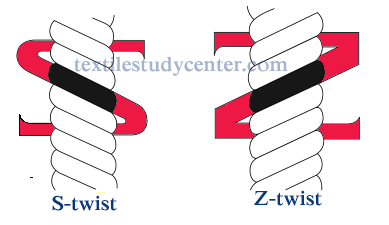# Study of Twist | Twist in yarn | Introduction of twist in yarn

17.76K
5#### Md Sohanur Rahman Sobuj

Co-founder , Admin & Author at Textile Study Center
Student of Bangladesh University of Textiles (BUTEX) Department: Apparel Engineering## Study of Twist in spinning | Twist in yarn | Introduction of twist in yarn

### Definition of twist:

The term “twist” defines the helicoidal or spiral configuration organized by the rotation of a yarn or of a bundle of fibers around their longitudinal axis. Or, Twist is the measurement of spiral turns given to a yarn in order to hold the constituent fibers or threads together.

Twist binds the fibers together and contributes strength to the spun yarn. The number of twist affects yarn and product performance and yarn cost.

Objects of twist:

To hold the staple fibers together.

To improve dynamometric properties (tenacity, elongation, modulus of elasticity) and
regularity.

To modify structural properties affecting tactile characteristics (touch) and appearance
(crêpe effect, covering power, lustre).

To reduce the tendency of textured yarns to rotate on their own axis and to form loops

The following four parameters are of importance when discussing twist in yarn:

Direction of twist

Twist level/ Amount of twist

Twist angle

Twist multiplier

### Direction of Twist:

In addition to the amount of twist in a yarn, the direction of the twist is also designated. There are two types:-

Z twist

S twist

### Z twist:

When a yarn is twisted in a clockwise direction, the fibers from a helical angle at the yarn surface. This angle conforms to the middle part of the letter “Z” and, therefore, is referred to as “Z” twist (Sometimes referred to as right-hand twist). Open end and ring spun yarn can be produce with “Z” twist. Z -twist is more common for weaving yarns.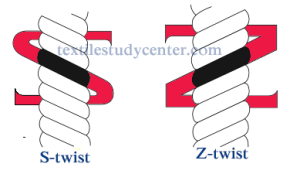### S twist:

When a yarn is twisted in an anticlockwise direction, the fibers from a helical angle at the yarn surface. This angle conforms to the middle part of the letter “S” and, therefore, is referred to as “S” twist ((Sometimes referred to as left-hand twist). Ring spun yarn (not open end yarn) can be produce with “S” twist.

In textile practics, it is customary to have Z-twist for warp yarns and S-twist for weft yarns.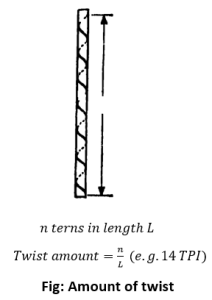### Twist Level / Amount of Twist:

The twist level (degree of twist) in a yarn is the number of turns of twist per unit length.Generally, filaments need a twist of 3-6 TPI while staples require a higher TPI of 10-20. The amount of twist varies with fiber length, yarn size, and its intended use.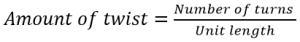Twist amount is expressed in-

TPI (Turns Per Inch)

TPM (Turns Per Meter)

TPCm (Turns Per Centimeter)

### Twist Angle

The twist angle θ is the angle between a tangent to the helix formed by the fiber and the yarn axis. By “unrolling” the surface layer we see that the fibers becomes the hypotenuse of a right-angled triangle.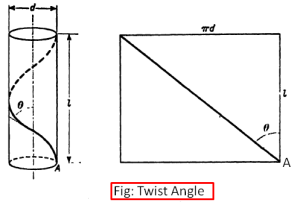Let the yarn diameter be D unit and let be the length of yarn occupied by one complete turn of twist. Then,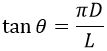The greater the diameter of the yarn, the larger is the angle produced by on turn of twist. As 1/L is equivalent to turns per unit length then: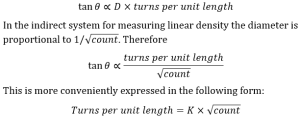This is the relation between yarn twist and yarn count where constant K is termed the

twist factor” or twist multiplier” and is directly proportional to the tangent of the twist angle.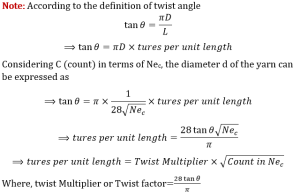Math: Calculate the twist angle of a spun cotton yarn twisted to give a twist factor of 7.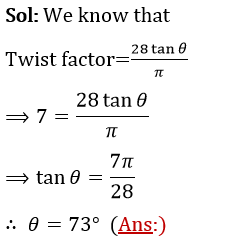### Twist multiplier:

A course yarns with 20 TPI has vastly different twist characteristics to a fine yarn with 20 TPI. By an expression known as the twist factor or twist multiplier.

Twist multiplier is an empirical parameter that has been establish by experiments and practice that the maximum strength of a yarn is obtained for a definite value of K. It is directly proportional to the tangent of the twist angle.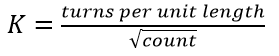Where K is the twist factor, also known as Twist Multiplier (TM).

Value of twist factor differs with each count system.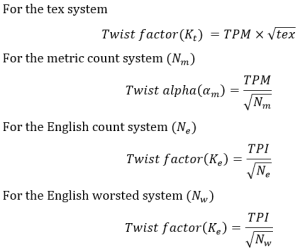Math: A combed cotton yarn Ne 50, has a twist of 1020 per meter. What is the English twist factor?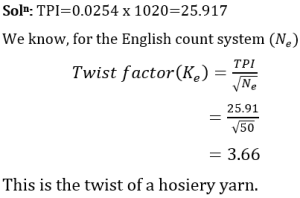Significance of the twist multiplier:

The twist multiplier is used to calculate the amount of TPI to be given to the cotton yarn during spinning. If yarns of soft feel and a pliable behavior is required twist factors around 3.0 is used while at the other example, hard twisted, stiff and twist lively yarns will have twist factors of around 6.0.

It describes the nature and character of a yarn i.e. characteristics like softness, smoothness, hardness, etc. of yarns. If yarns of different counts were spun with the same twist factor, their characteristics would be similar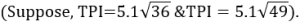Conversely, if yarns of the same count differed in twist factor, they would have markedly different characteristics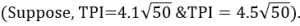Twist for various subsequent process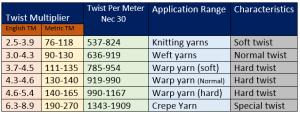Function of twist in yarn structure:

The main function of twist is to be bind the fibers together and helps to keep them in their respective positions. It thus gives coherence to the yarn.

Without twist a strand of fibers has very little strength and in the first instance a yarn must have sufficient tensile strength to withstand the stresses of preparation and fabric manufacture.

The main function of twist is to give coherence to the yarn. In order to develop strength in a twisted strand of discontinuous fibers (e.g. cotton yarn) and so resist breakage, the individual fibers must grip each other when the strand is stressed. This cohesion arises mainly from twist, which presses the fibers together as the stretching force is applied and so developing friction between adjacent fibers.

Twist is also used to bring about novel effects that are prominently visible when the yarn is converted to fabric. This is achieved primarily by having a combination of yarns with different twist levels and twist direction in the fabric.

Factors Affecting Twist:

The count of yarn to be spun-the twist level in a yarn is proportional to the square root of the yarn count.

The quality of cotton used-all other parameters remaining the same, longer fibers require less twist than the shorter ones.

The use to which the yarn is put-is the yarn meant to be used as warp yarn, weft yarn, knitting yarn or any other yarn.

The fineness of the fiber being spun-the finer and longer staple cottons needs a lower twist for the same count compared to shorter and coarser cottons.

The kind of machine in which the yarn will subsequently be used-the twist in yarns used for weaving on power looms and automatic looms will be higher than that used for hosiery.

The softness of the fabric into which the yarn is to be converted-yarns required for soft-surfaced fabrics are given only a slack twist.

The smoothness of the fabric into which the yarn is to be converted-yarn required for smooth-surfaced fabrics are given many twist per inch. They are hard twisted yarns and they give strength, smoothness, elasticity and some crease-resistance of fabrics.

Any other special attribute of the fabric into which the yarn is to be converted-yarns required for crepe fabrics with rough, pebbly or crinkled surfaces are given a maximum amount of twist. The crepe yarns also confer enhanced cease resistance to the fabric.

(17756)#### Md Sohanur Rahman Sobuj

Co-founder , Admin & Author at Textile Study Center
Student of Bangladesh University of Textiles (BUTEX) Department: Apparel Engineering#### Latest posts by Md Sohanur Rahman Sobuj (see all)Close
Share via